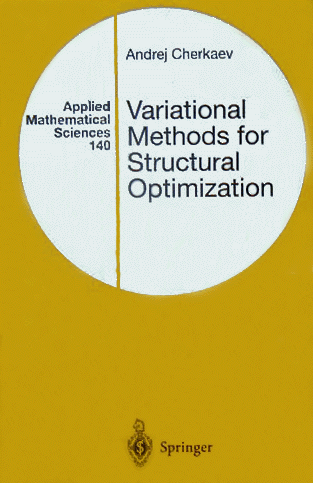This is the Web site for the bookVariational methods for Structural Optimization

by Andrej Cherkaev

Springer Verlag, NY 2000.

@Book{Cherkaev:2000:VMS,
author =       "Andrej Cherkaev",
title =        "Variational Methods for Structural Optimization",
publisher =    "Springer-Verlag",
address =      "Berlin / Heidelberg / New York / etc.",
year =         "2000",
ISBN =         "ISBN 0-387-98462-3",
LCCN = "QA1 .A647 v.140; TA658.8 .C53 2000",
pages =        "xii + 627",}

|| Book's homepage || List of Chapters || Springer site and Order || Order from Amazon || Order from Barnes & Noble || Andrej Cherkaev Homepage ||

Focus

The book describes mathematical foundations of structural optimization. Optimal  structures adapt the construction to its goal and to loading conditions. Mathematically, the problem is formulated as a variational problem. It possesses non-stable solutions: the optimization of materials collocations results in a system of patterns or in optimal microstructures. These occur if the Lagrangian lacks the convexity or the quasiconvexity. To relax the problem, a quasiconvex envelope of the Lagrangian must be built; the book discusses methods for obtaining quasiconvex envelopes.

First chapters introduce  unstable variational problems and conductivity and elasticity of inhomogeneous media. Then the structural optimization problems are formulated. The tools for bounding of the quasiconvex envelope are described. Those are special necessary conditions of optimality, minimal extensions, minimizing sequences, and the Translation method of sufficient conditions. Special attention is paid to the problem of G-closure, that is to description of all possible material characteristics of a composites with arbitrary geometry. The methods are described to determine geometry of optimal structures and their homogenized behavior. Other topics include  min-max formulation, and stability of optimal solutions. Many examples of structural optimization are discussed.

The book is addressed to students and researchers is applied mathematics, mechanics, and material sciences. Its challenge is to expose the foundations of structural optimization in simple terms to make them available for practical use and adaptable to specific models. A number of problems is listed in the end of the chapters.

The style is informal: the usual conflict between mathematical rigor and the physical clarity usually is solved in favor of the last one. The simplest possible mathematical tools are used. New mathematical theories are not introduced if the goals can be achieved using a simpler representation. The upper bound of the complexity is given by the Ockham's Razor Principle: "Pluralitas non est ponenda sine neccesitate" -- entities must not be multiplied beyond what is necessary. The lower bound comes from the Einstein's warning " Everything should be made as simple as possible, but not simpler."